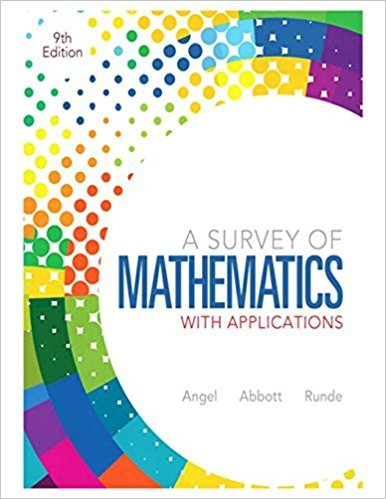×
×

# Solutions for Chapter 11.5: Consumer Mathematics## Full solutions for A Survey of Mathematics with Applications | 9th Edition

ISBN: 9780321759665Solutions for Chapter 11.5: Consumer Mathematics

Solutions for Chapter 11.5
4 5 0 349 Reviews
23
5
##### ISBN: 9780321759665

Since 31 problems in chapter 11.5: Consumer Mathematics have been answered, more than 79937 students have viewed full step-by-step solutions from this chapter. A Survey of Mathematics with Applications was written by and is associated to the ISBN: 9780321759665. This expansive textbook survival guide covers the following chapters and their solutions. Chapter 11.5: Consumer Mathematics includes 31 full step-by-step solutions. This textbook survival guide was created for the textbook: A Survey of Mathematics with Applications, edition: 9.

Key Math Terms and definitions covered in this textbook
• Affine transformation

Tv = Av + Vo = linear transformation plus shift.

• Associative Law (AB)C = A(BC).

Parentheses can be removed to leave ABC.

• Block matrix.

A matrix can be partitioned into matrix blocks, by cuts between rows and/or between columns. Block multiplication ofAB is allowed if the block shapes permit.

• Cramer's Rule for Ax = b.

B j has b replacing column j of A; x j = det B j I det A

• Determinant IAI = det(A).

Defined by det I = 1, sign reversal for row exchange, and linearity in each row. Then IAI = 0 when A is singular. Also IABI = IAIIBI and

• Diagonal matrix D.

dij = 0 if i #- j. Block-diagonal: zero outside square blocks Du.

• Free columns of A.

Columns without pivots; these are combinations of earlier columns.

• Gram-Schmidt orthogonalization A = QR.

Independent columns in A, orthonormal columns in Q. Each column q j of Q is a combination of the first j columns of A (and conversely, so R is upper triangular). Convention: diag(R) > o.

• Hankel matrix H.

Constant along each antidiagonal; hij depends on i + j.

• Linear transformation T.

Each vector V in the input space transforms to T (v) in the output space, and linearity requires T(cv + dw) = c T(v) + d T(w). Examples: Matrix multiplication A v, differentiation and integration in function space.

• Lucas numbers

Ln = 2,J, 3, 4, ... satisfy Ln = L n- l +Ln- 2 = A1 +A~, with AI, A2 = (1 ± -/5)/2 from the Fibonacci matrix U~]' Compare Lo = 2 with Fo = O.

• Multiplicities AM and G M.

The algebraic multiplicity A M of A is the number of times A appears as a root of det(A - AI) = O. The geometric multiplicity GM is the number of independent eigenvectors for A (= dimension of the eigenspace).

• Orthogonal subspaces.

Every v in V is orthogonal to every w in W.

• Partial pivoting.

In each column, choose the largest available pivot to control roundoff; all multipliers have leij I < 1. See condition number.

• Reduced row echelon form R = rref(A).

Pivots = 1; zeros above and below pivots; the r nonzero rows of R give a basis for the row space of A.

• Right inverse A+.

If A has full row rank m, then A+ = AT(AAT)-l has AA+ = 1m.

• Singular matrix A.

A square matrix that has no inverse: det(A) = o.

• Symmetric factorizations A = LDLT and A = QAQT.

Signs in A = signs in D.

• Triangle inequality II u + v II < II u II + II v II.

For matrix norms II A + B II < II A II + II B II·

• Vector space V.

Set of vectors such that all combinations cv + d w remain within V. Eight required rules are given in Section 3.1 for scalars c, d and vectors v, w.

×